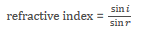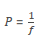# unit 3

?
• Created by: jonknees
• Created on: 25-03-15 15:59

## X-rays

a) X-rays are part of the electromagnetic spectrum. They have a very short wavelength and cause ionisation.

Properties of X-rays include:

• they affect a photographic film in the same way as light
• they are absorbed by metal and bone
• they are transmitted by soft tissue
• their wavelength is of the same order of magnitude as the diameter of an atom.

b) X-rays can be used to diagnose and treat some medical conditions.

c) Precautions to be taken when X-ray machines and CT scanners are in use.

1 of 14

## Ultrasound

a) Electronic systems can be used to produce ultrasound waves, which have a frequency higher than the upper limit of hearing for humans.

human hearing is about 20 Hz to 20 000 Hz.

b) Ultrasound waves are partially reflected when they meet a boundary between two different media. The time taken for the reflections to reach a detector can be used to determine how far away such a boundary is.

c) Calculation of the distance between interfaces in various media.

s= v x t

• s is distance in metres, m
• v is speed in metres per second, m/s
• t is time in seconds, s

d) Ultrasound waves can be used in medicine.

2 of 14

## Lenses

a) Refraction is the change of direction of light as it passes from one medium to another.

b) A lens forms an image by refracting light.

c) In a convex or converging lens, parallel rays of light are brought to a focus at the principal focus. The distance from the lens to the principal focus is called the focal length.i is the angle of incidence

• r is the angle of refraction

3 of 14

## lenses part 2

d) The nature of an image is defined by its size relative to the object, whether it is upright or inverted relative to the object and whether it is real or virtual.

e) The nature of the image produced by a converging lens for an object placed at different distances from the lens.

f) The use of a converging lens as a magnifying glass.

g) The nature of the image produced by a concave or diverging lens.

h) The construction of ray diagrams to show the formation of images by converging and diverging lenses.

i) The magnification produced by a lens is calculated using the equation:

magnification = image height x object height

4 of 14

## The eye

a) The structure of the eye. The structure of the eye is limited to:

• retina
• lens
• cornea
• pupil /iris
• ciliary muscle
• suspensory ligaments.

b) Correction of vision using convex and concave lenses to produce an image on the retina:

• long sight, caused by the eyeball being too short, or the eye lens being unable to focus
• short sight, caused by the eyeball being too long, or the eye lens being unable to focus.

c) Range of vision. The eye can focus on objects between the near point and the far point.

5 of 14

## The eye part 2

d) Comparison between the structure of the eye and the camera.

e) The power of a lens is given by:P is power in dioptres, D

• f is focal length in metres, m

f) The focal length of a lens is determined by:

• the refractive index of the material from which the lens is made, and
• the curvature of the two surfaces of the lens.
6 of 14

## Other applications using light

a) Total internal reflection and critical angle.

refractive index=1sinc

c is the critical angle

b) Visible light can be sent along optical fibres.

Examples of use should include the endoscope for internal imaging.

c) The laser as an energy source for cutting, cauterising and burning.

7 of 14

## centre of mass

a) The centre of mass of an object is that point at which the mass of the object may be thought to be concentrated.

b) If freely suspended, an object will come to rest with its centre of mass directly below the point of suspension.

c) The centre of mass of a symmetrical object is along the axis of symmetry.

d) For a simple pendulum:

T=1 / f

• T is periodic time in seconds, s
• f is frequency in hertz, Hz

e) The time period depends on the length of a pendulum.

Applications of the pendulum should include simple fairground and playground rides.

8 of 14

## moments

a) The turning effect of a force is called the moment.

b) The size of the moment is given by the equation: M=F×d

• M is the moment of the force in newton-metres, Nm
• F is the force in newtons, N
• d is the perpendicular distance from the line of action of the force to the pivot in metres, m

c) If an object is not turning, the total clockwise moment must be exactly balanced by the total anticlockwise moment about any pivot.

e) Ideas of simple levers.

Limited to levers as force multipliers.

f) If the line of action of the weight of an object lies outside the base of the object there will be a resultant moment and the body will tend to topple.

9 of 14

## Circular motion

a) When an object moves in a circle it continuously accelerates towards the centre of the circle. This acceleration changes the direction of motion of the body, not its speed.

b) The resultant force causing this acceleration is called the centripetal force and is always directed towards the centre of the circle.

c) The centripetal force needed to make an object perform circular motion increases as:

• the mass of the object increases
• the speed of the object increases
• the radius of the circle decreases.
10 of 14

## the motor effect

a) When a current flows through a wire a magnetic field is produced around the wire.

b) The motor effect and its use.

c) The size of the force can be increased by:

• increasing the strength of the magnetic field
• increasing the size of the current.

d) The conductor will not experience a force if it is parallel to the magnetic field.

e) The direction of the force is reversed if either the direction of the current or the direction of the magnetic field is reversed

.11 of 14

## Transformers

a) If an electrical conductor 'cuts' through a magnetic field a potential difference is induced across the ends of the conductor.

b) If a magnet is moved into a coil of wire a potential difference is induced across the ends of the coil.

c) The basic structure of the transformer.

d) An alternating current in the primary coil produces a changing magnetic field in the iron core and hence in the secondary coil. This induces an alternating potential difference across the ends of the secondary coil.

e) In a step-up transformer the potential difference across the secondary coil is greater than the potential difference across the primary coil.

f) In a step-down transformer the potential difference across the secondary coil is less than the potential difference across the primary coil.

g) The potential difference across the primary and secondary coils of a transformer are related by the equation:

VpVs=npns

• Vp is the potential difference across the primary coil in volts, V
• Vs is the potential difference across the secondary coil in volts, V
• np is the number of turns on the primary coil
• ns is the number of turns on the secondary coil

h) If transformers are assumed to be 100% efficient, the electrical power output would equal the electrical power input.

Vp×Ip=Vs×Is

• Vp is the potential difference across the primary coil in volts, V
• Ip is the current in the primary coil in amperes (amps), A
• Vs is the potential difference across the secondary coil in volts, V
• Is is the current in the secondary coil in amperes (amps), A

i) Switch mode transformers operate at a high frequency, often between 50 kHz and 200 kHz.

j) Switch mode transformers are much lighter and smaller than traditional transformers working from a 50 Hz mains supply.

k) Switch mode transformers use very little power when they are switched on but no load is applied.

12 of 14

## Transformers part 2

g) The potential difference across the primary and secondary coils of a transformer are related by the equation:

VpVs=npns

• Vp is the potential difference across the primary coil in volts, V
• Vs is the potential difference across the secondary coil in volts, V
• np is the number of turns on the primary coil
• ns is the number of turns on the secondary coil
13 of 14

## Transformers part 3

h) If transformers are assumed to be 100% efficient, the electrical power output would equal the electrical power input.

Vp×Ip=Vs×Is

• Vp is the potential difference across the primary coil in volts, V
• Ip is the current in the primary coil in amperes (amps), A
• Vs is the potential difference across the secondary coil in volts, V
• Is is the current in the secondary coil in amperes (amps), A

i) Switch mode transformers operate at a high frequency, often between 50 kHz and 200 kHz.

j) Switch mode transformers are much lighter and smaller than traditional transformers working from a 50 Hz mains supply.

k) Switch mode transformers use very little power when they are switched on but no load is applied.

14 of 14